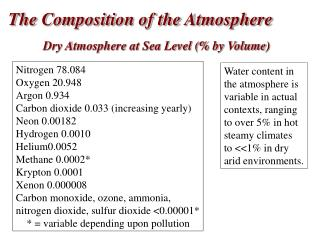DownloadDownload PresentationThe Composition of the Atmosphere

# The Composition of the Atmosphere

Download Presentation## The Composition of the Atmosphere

- - - - - - - - - - - - - - - - - - - - - - - - - - - E N D - - - - - - - - - - - - - - - - - - - - - - - - - - -
##### Presentation Transcript

1. The Composition of the Atmosphere Dry Atmosphere at Sea Level (% by Volume) Nitrogen 78.084 Oxygen 20.948 Argon 0.934 Carbon dioxide 0.033 (increasing yearly) Neon 0.00182 Hydrogen 0.0010 Helium0.0052 Methane 0.0002* Krypton 0.0001 Xenon 0.000008 Carbon monoxide, ozone, ammonia, nitrogen dioxide, sulfur dioxide <0.00001* * = variable depending upon pollution Water content in the atmosphere is variable in actual contexts, ranging to over 5% in hot steamy climates to <<1% in dry arid environments.

2. Pressure 760 mm (at sea level) Hg Atmospheric pressure is measured with a barometer If a tube is inserted into a container of mercury open to the atmosphere, the mercury will rise 760 mm up the tube (at sea level).

3. The Gas Laws The Pressures-Volume Relationship: Boyle’s Law P is inversely proportional to V (at constant T) Mathematically: • A plot of V versus P is a hyperbola. • Similarly, a plot of V versus 1/P must be a straight line passing through the origin.

4. The Gas Laws The Temperature-Volume Relationship: Charles’ Law (We know that hot air balloons expand when they are heated.) The volume of a fixed quantity of gas at constant pressure increases as the temperature increases. Mathematically: • A plot of V versus T is a straight line. • When T is measured in C, the intercept on the temperature axis is -273.15C. • We define absolute zero, 0 K = -273.15C.

5. 22.4 L = constant  1 mole at a 1 atm and 273 K V = constant  n at a constant P and T

6. Kinetic-Molecular Theory (KMT) • Theory of moving molecules, explains gas behavior, gives us an understanding of temperature and pressure effects on the molecular level. • Assumptions: • (1) Gases consist of a large number of molecules in constant random motion. • (2) Volume of individual gas particles is negligible compared to volume of container (point particles). • (3) Intermolecular forces (forces between gas molecules) are negligible. • (4) All particle collisions with walls are elastic (no loss of energy). • (5) All energy and momenta conserved.

7. Kinetic-Molecular Theory (KMT) Pressure of a gas results from the number of collisions per unit time on the walls of container. The energy of gas molecules depends only on temperature. Thus, heavier molecules move more slowly (at the same temperature).

8. Acids and Bases: A Brief Review Classical Acids: Taste sour Donate H+ (called “H-plus” or “proton”) Turn litmus red Generally formed from H-Z, where Z = nonmetal Classical Bases: Taste bitter and feel soapy. Donate OH- (called “O-H-minus” or “hydroxide”) Turn litmus blue Generally formed from MOH, where M = metal Neutralization: Acid + Base  Salt + water H-Z + MOH  MZ + HOH H+ in water is actually in the form of H3O+, “hydronium”

9. Strong and Weak Acids and Bases Strong acids: completely ionized in water: HCl, HNO3, H2SO4 (also HBr, HI) Strong bases: completely ionized in water: MOH, where M = alkali M(OH)2, where M = alkaline earth Weak acids: incompletely ionized in water: any acid that is not strong - acetic acid, etc. Ka is finite. Weak bases: incompletely ionized in water: any base that is not strong – NH3, etc. Kb is finite.

10. The pH Scale Conc. Drano Battery acid